Courses
Courses for Kids
Free study material
Offline Centres
More

# What is a Straight LineLast updated date: 02nd Dec 2023
Total views: 99k
Views today: 0.99k## Introduction

The line definition, as stated by Euclid, basically states that a straight line connects any two given points and can be extended forever in both directions. The shortest distance between two points is a straight line.

A straight line is a continuous, one-dimensional shape with no breadth. It consists of an infinite number of points connected on either side of a point. It might be inclined, vertical, or horizontal. Any angle we draw between two locations along a straight line will always be a 180-degree angle.

## What is a Straight Line?

A straight line has no curvature. A straight line can also be drawn between any two points, but its endpoints must stretch into the infinite. When two points, A (x1, y1) and B (x2, y2) are connected by the shortest path possible, a straight line is a result. We see straight lines in our day-to-day life, such as railway tracks, Roads, and Bridges.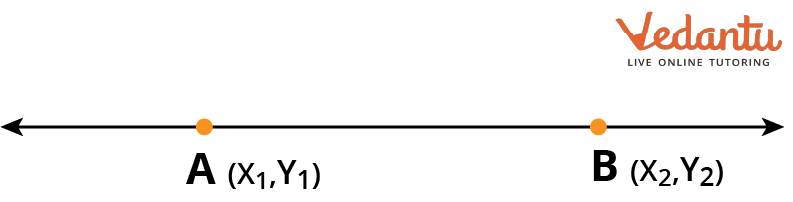Straight Line

## Types of Straight Lines

There are several kinds of straight lines. The alignment of the straight lines is typically used to categorise them. The angle they make with either the x-axis or the y-axis is referred to as their alignment. Straight lines can be classified into the following categories based on their alignment:

• Horizontal Lines

• Vertical Lines

• Oblique/Inclined Lines

### Horizontal Lines

Horizontal lines are parallel to the x-axis or perpendicular to the y-axis when drawn horizontally. They intersect the x-axis at an angle of 0° or 180° and the y-axis at an angle of 90° or 270°. This line does not touch any point on the X-axis. All the points on this line will have the same Y-coordinate.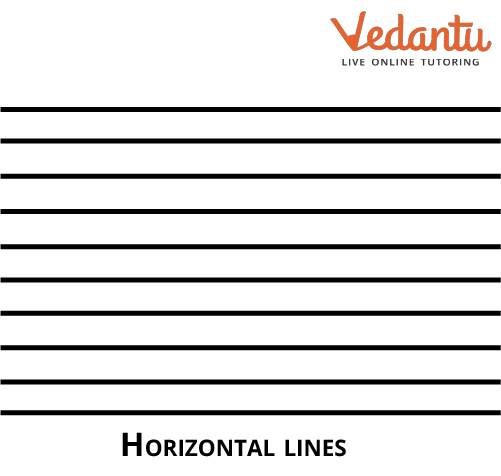Horizontal Line

### Vertical Lines

Vertical lines are drawn vertically and parallel to the y-axis or perpendicular to the x-axis. They intersect the x-axis at an angle of 90° or 270° and the y-axis at an angle of 0° or 180°. All the points on this line will have the same X-coordinate.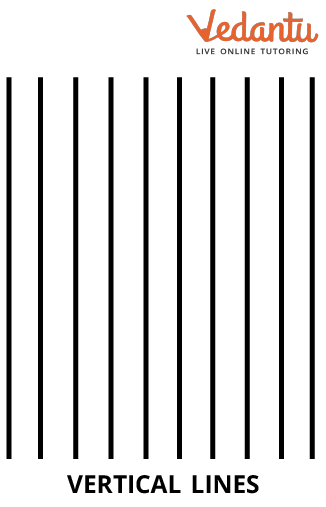Vertical Lines

### Oblique/Inclined Lines

Oblique or slanting lines are those that are drawn at an angle other than 0°, 90°, 180°, 270°, or 360° with the horizontal or vertical lines. Oblique or inclined lines are straight lines that are neither horizontal nor vertical.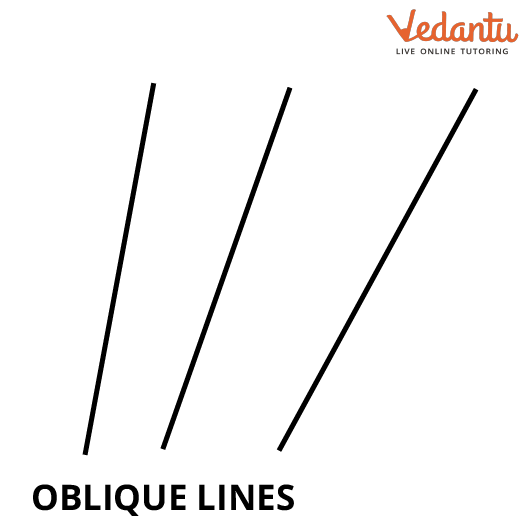Oblique Lines

## Properties of Straight Lines

The properties of straight lines are illustrated below:

• A straight line can go on forever. The distance between the line's two extreme points is impossible to measure.

• It has no volume and no areas. However, its length is unlimited.

• It is a figure with only one dimension.

• A single point can be traversed by an endless number of lines, but only one single line can pass between two points.

## Equation of a Straight Line

An equation of a straight line is a linear equation. Depending on the known variables, angles, and constants, a straight line on a plane can be represented in a variety of ways. The direction of a straight line is determined by its slope, which also indicates how steep the line is. It is calculated as the difference in y coordinates/difference in x coordinates and is also referred to as rise over run. There are several ways to write a straight-line equation.

### General Equation of a Straight Line

The general formula for a straight line is $ax + by + c = 0$, where

The slope will be $\dfrac{{ - a}}{b}$.

Here, a, b, and c are constants, and x, and y are variables.

### Slope Point Form:

The idea of calculating a line's slope from two points is the basis of the slope-point form. A straight line going through the point $\left( {{x_1},{y_1}} \right)$ and having a slope of $m = \tan \theta$, where $\theta$ is the angle produced by the line with the positive x-axis is given as $(y - {y_1}) = m(x - {x_1})$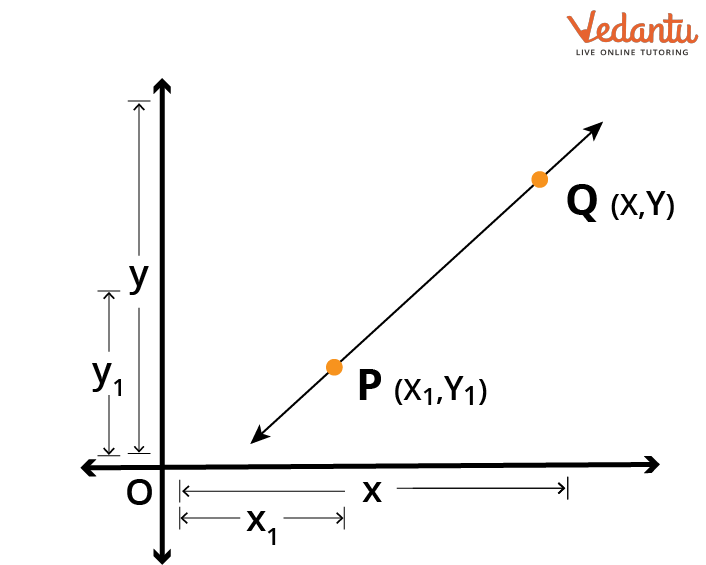Slope point form

### Two-Point Form:

When two points along a line are known, the equation of the line may be found using the two-point form. Given by is a straight line that connects the coordinates $\left( {{x_1},{y_1}} \right)$ and $\left( {{x_2},{y_2}} \right)$. The two-point form is: $y - {y_1} = \dfrac{{{y_2} - {y_1}}}{{{x_2} - {x_1}}}(x - {x_1})$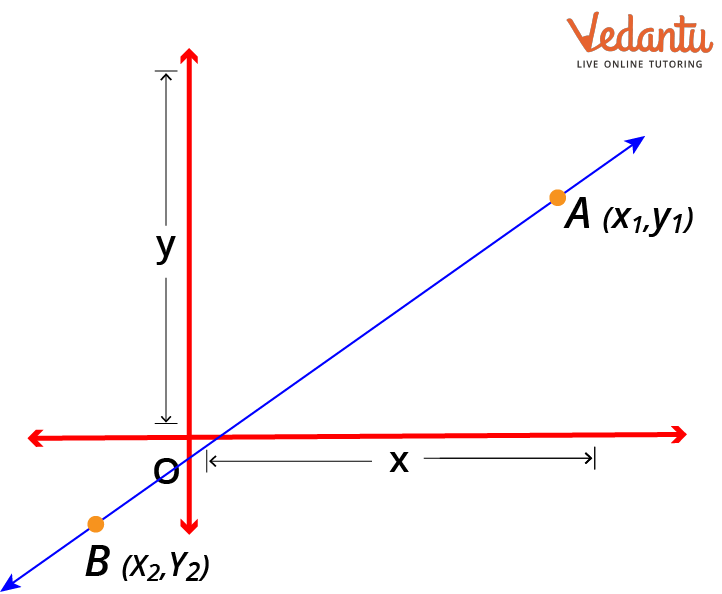Two-point form

### Intercept Form:

The point where the graph crosses the axes is known as the intercept. The point where the graph meets the x-axis is known as the x-intercept if the graph crosses the x-axis. The point at which a graph contacts the y-axis is referred to as the y-intercept. The intercept form of a straight line with point A on the x-axis and point B on the y-axis, as seen in the picture below, is expressed as a straight line with the x-intercept as a and y-intercept as b. The intercept form is: $\dfrac{x}{a} + \dfrac{y}{b} = 1$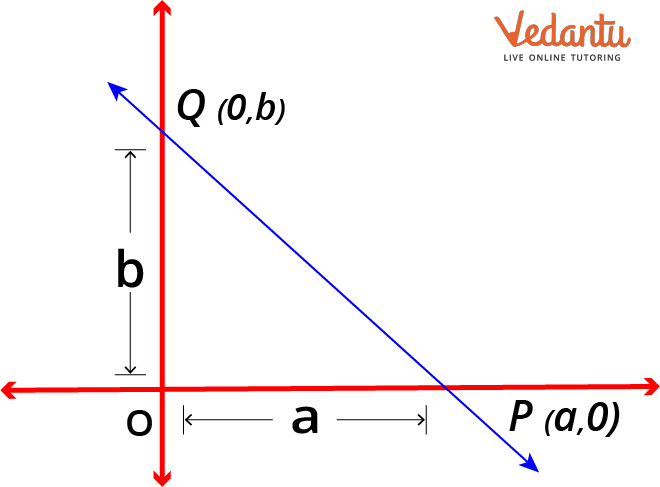Intercept form

## Straight Line Problems:

Let us look at some straight line questions and answers or Problems on Straight Lines:

Example 1: What will be the equation of the line passing through the points (2,3) and (5,4)?

Solution: To find the equation of a line passing through the points (2,3) and (5,4), we will use the two-point form:

$y - {y_1} = \dfrac{{{y_2} - {y_1}}}{{{x_2} - {x_1}}}(x - {x_1})$

Substituting the values, we get

$\begin{array}{l}y - 3 = \dfrac{{4 - 3}}{{5 - 2}}(x - 2)\\3y - 9 = x - 2\\3y - x - 7 = 0\end{array}$

Therefore, the equation of a line is $3y - x - 7 = 0$.

Example 2: What is the equation of the line which has a slope of 3 and passes through a point (2,5)?

Solution: To find the equation of the line with slope 3 and passing through a point (2,5) we will use slope point form:

$(y - {y_1}) = m(x - {x_1})$

Substituting the values,

$\begin{array}{l}y - 5 = 3(x - 2)\\y - 5 = 3x - 6\\y - 3x + 1 = 0\end{array}$

### Conclusion:

We now have a basic understanding of the line and its key components due to this article. We will be able to apply the principles to real-world applications and discover more fascinating facts about the line using the many sorts of lines that have been discussed in this article.

## FAQs on What is a Straight Line

1: Why do we need straight lines in our daily lives?

In performing research and creating the government budget, straight-line graphs are used. To check whether our body weight is acceptable for our height, straight-line graphs are used.

2: Who invented the straight line?

When Euclid first formalised geometry in the Elements, he defined a general line (straight or curved) to be "breadthless length", with a straight line being a line "which lies evenly with the points on itself".

3: What is the origin of the line?

The first step to finding the origin of a line is identifying if it passes through c, the intercept of the y and x-axis. If it does, then the point that it passes through is the origin of that line. In other words, the point when the coordinates of a line at either y or x-axis are equal to zero is the origin of that line.